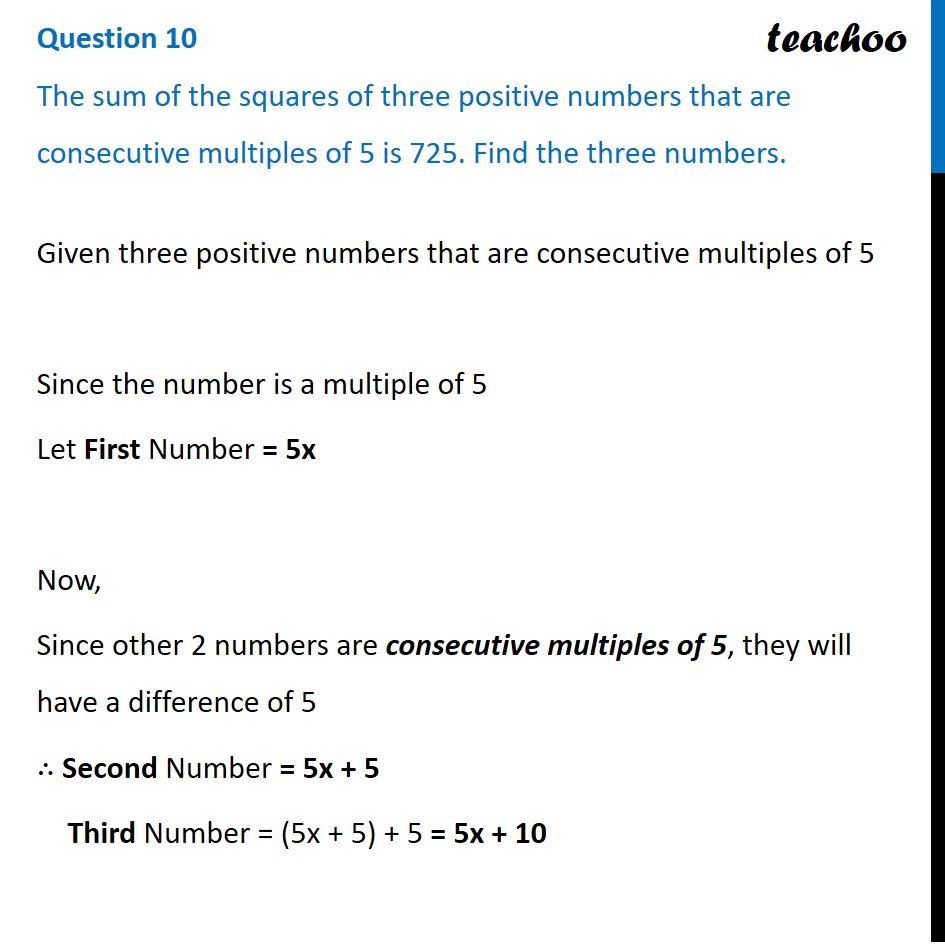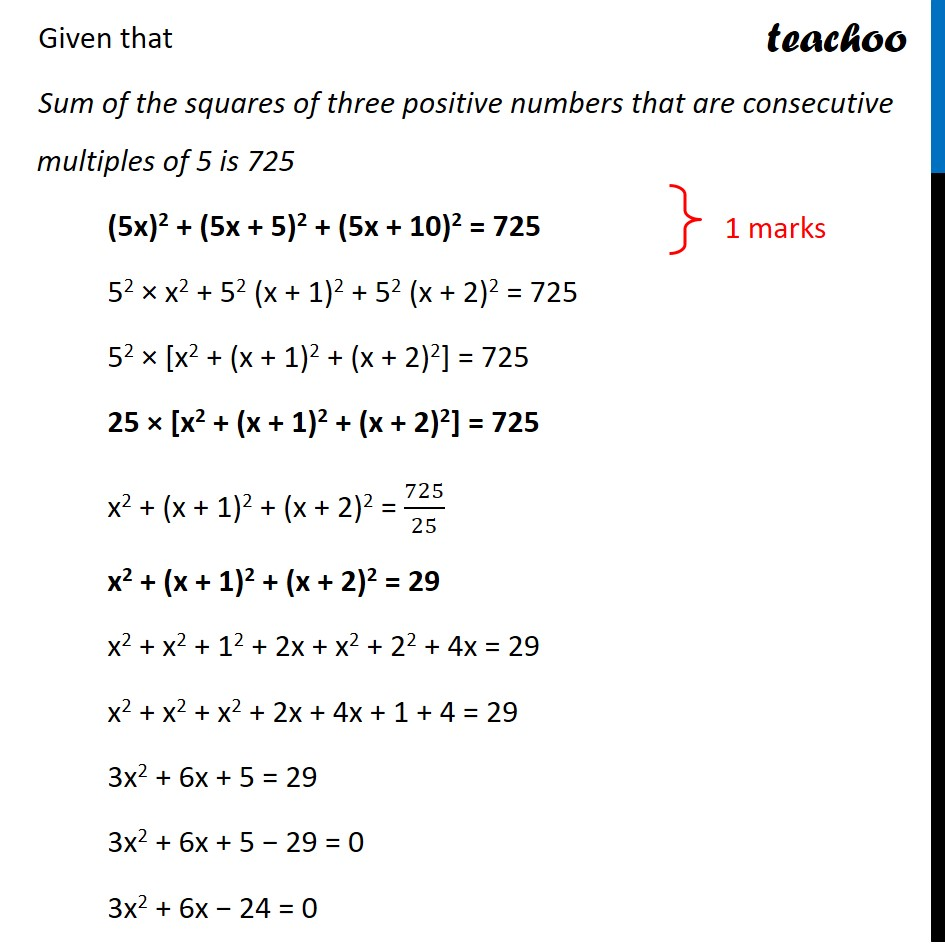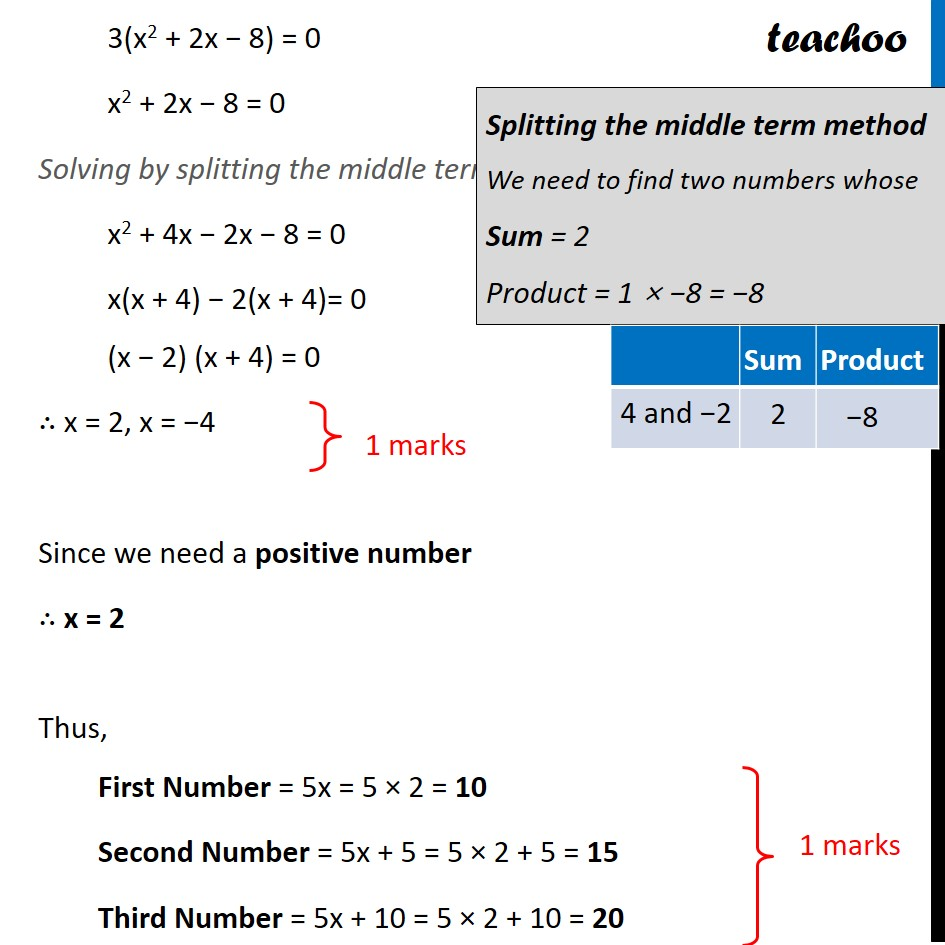CBSE Class 10 Sample Paper for 2022 Boards - Maths Basic [Term 2]

Class 10
Solutions of Sample Papers for Class 10 Boards

## The sum of the squares of three positive numbers that are consecutive multiples of  5 is 725. Find the three numbers.

This question is similar to Ex 4.2, 4 Chapter 4 Class 10 - Quadratic EquationsLearn in your speed, with individual attention - Teachoo Maths 1-on-1 Class

### Transcript

Question 10 The sum of the squares of three positive numbers that are consecutive multiples of 5 is 725. Find the three numbers. Given three positive numbers that are consecutive multiples of 5 Since the number is a multiple of 5 Let First Number = 5x Now, Since other 2 numbers are consecutive multiples of 5, they will have a difference of 5 ∴ Second Number = 5x + 5 Third Number = (5x + 5) + 5 = 5x + 10 Given that Sum of the squares of three positive numbers that are consecutive multiples of 5 is 725 (5x)2 + (5x + 5)2 + (5x + 10)2 = 725 52 × x2 + 52 (x + 1)2 + 52 (x + 2)2 = 725 52 × [x2 + (x + 1)2 + (x + 2)2] = 725 25 × [x2 + (x + 1)2 + (x + 2)2] = 725 x2 + (x + 1)2 + (x + 2)2 = 725/25 x2 + (x + 1)2 + (x + 2)2 = 29 x2 + x2 + 12 + 2x + x2 + 22 + 4x = 29 x2 + x2 + x2 + 2x + 4x + 1 + 4 = 29 3x2 + 6x + 5 = 29 3x2 + 6x + 5 − 29 = 0 3x2 + 6x − 24 = 0 3(x2 + 2x − 8) = 0 x2 + 2x − 8 = 0 Solving by splitting the middle term x2 + 4x − 2x − 8 = 0 x(x + 4) − 2(x + 4)= 0 (x − 2) (x + 4) = 0 ∴ x = 2, x = −4 Since we need a positive number ∴ x = 2 Thus, First Number = 5x = 5 × 2 = 10 Second Number = 5x + 5 = 5 × 2 + 5 = 15 Third Number = 5x + 10 = 5 × 2 + 10 = 20